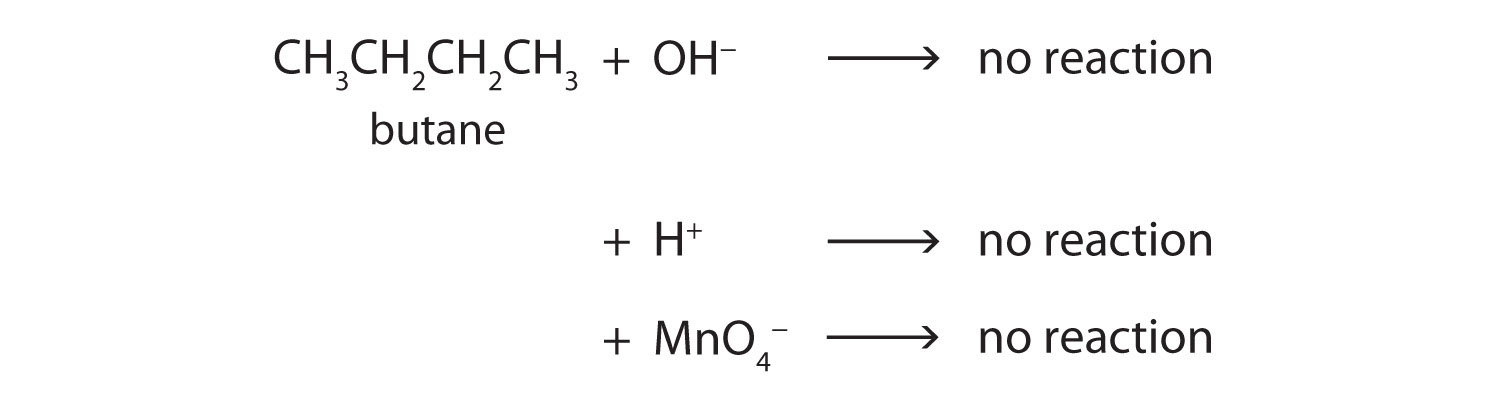# 12.7: Chemical Properties of Alkanes

Skills to Develop

• To identify the main chemical properties of alkanes.

Alkane molecules are nonpolar and therefore generally do not react with ionic compounds such as most laboratory acids, bases, oxidizing agents, or reducing agents. Consider butane as an example:Neither positive ions nor negative ions are attracted to a nonpolar molecule. In fact, the alkanes undergo so few reactions that they are sometimes called paraffins, from the Latin parum affinis, meaning “little affinity.”

Two important reactions that the alkanes do undergo are combustion and halogenation. Nothing happens when alkanes are merely mixed with oxygen ($$O_2$$) at room temperature, but when a flame or spark provides the activation energy, a highly exothermic combustion reaction proceeds vigorously. For methane (CH4), the reaction is as follows:

$CH_4 + 2O_2 \rightarrow CO_2 + 2H_2O + \text{heat} \label{12.7.1}$

If the reactants are adequately mixed and there is sufficient oxygen, the only products are carbon dioxide ($$CO_2$$), water ($$H_2O$$), and heat—heat for cooking foods, heating homes, and drying clothes. Because conditions are rarely ideal, however, other products are frequently formed. When the oxygen supply is limited, carbon monoxide ($$CO$$) is a by-product:

$2CH_4 + 3O_2 \rightarrow​ 2CO + 4H_2O\label{12.7.2}$

This reaction is responsible for dozens of deaths each year from unventilated or improperly adjusted gas heaters. (Similar reactions with similar results occur with kerosene heaters.)

Alkanes also react with the halogens chlorine ($$Cl_2$$) and bromine ($$Br_2$$) in the presence of ultraviolet light or at high temperatures to yield chlorinated and brominated alkanes. For example, chlorine reacts with excess methane ($$CH_4$$) to give methyl chloride ($$CH_3Cl$$).

$CH_4 + Cl_2 \rightarrow​ CH_3Cl + HCl\label{12.7.3}$

With more chlorine, a mixture of products is obtained: CH3Cl, CH2Cl2, CHCl3, and CCl4. Fluorine ($$F_2$$), the lightest halogen, combines explosively with most hydrocarbons. Iodine ($$I_2$$) is relatively unreactive. Fluorinated and iodinated alkanes are produced by indirect methods.

## Concept Review Exercises

1. Why are alkanes sometimes called paraffins?
2. Which halogen reacts most readily with alkanes? Which reacts least readily?

1. Alkanes do not react with many common chemicals. They are sometimes called paraffins, from the Latin parum affinis, meaning “little affinity.”
2. most readily: $$F_2$$; least readily: $$I_2$$

## Key Takeaway

• Alkanes react with oxygen (combustion) and with halogens (halogenation).

## Exercises

1. Why do alkanes usually not react with ionic compounds such as most laboratory acids, bases, oxidizing agents, or reducing agents?
2. Write an equation for the complete combustion of methane ($$CH_4$$), the main component of natural gas).
3. What is the most important reaction of alkanes?
4. Name some substances other than oxygen that react readily with alkanes.